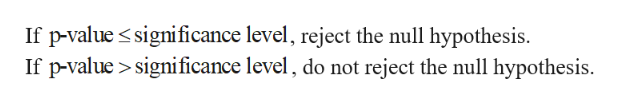# 1. Identify the parameter of interest. Describe it in the context of thesituation.2. Determine null value and state null hypothesis.3. State the appropriate alternative hypothesis.4. Give the formula for the computed value of the test statistic.5. State the rejection region for the selected level of significance, a.6. Compute sample quantities (if necessary), substitute into formula for thetest statistic value, and compute that value (z in this case).7. Decide whether or not the null hypothesis should be rejected and state theconclusion in the context of the original problem. (Either "reject" or "fail toreject the null hypothesis; never "accept" the null hypothesis. Next,explain what this means in terms of your specific situation, e.g., "concludethat the mean sulfur content is greater than 1.50%.") For the problems below, when asked to conduct the hypothesis test, please use the seven-stepprocess we have been using in class. Also, in step 7, it is very important to be clear in yourconclusion. There are three parts to this: evidence from your hypothesis test, the decision to rejector fail to reject the null hypothesis, and the conclusion in the context of the problem. For example,referring to the first example we did in Chapter 10, we stated the following"-1.03 is not less than -1.96, nor is it greater than +1.96, so we fail to reject Ho: Hi - 2 0, andconclude that there is no difference in mean yield strength between the two types of roof bolts."(Here, the evidence is-1.03 is not less than -1.96, nor is it greater than +1.96The decision isWe fail to reject Ho: H1-2 = 0The conclusion in the context of the problem isWe conclude that there is no difference in mean yield strength between the two types ofroof bolts)A study was performed to determine whether men and women differ in repeatability in6.assembling components on printed circuit boards. Random samples of 25 men and 21 women wereselected, and each subject assembled the units. The two sample standard deviations of assemblytime were smen = 0.98 minutes and swomen 1.02 minutes.(a) Use the seven-step hypothesis test that we have been using in class to determine if men andwomen differ in repeatability for this assembly task. Use a = 0.02 and state any necessaryassumptions about the underlying distribution of the data. Do not use the P-Value.(b) Determine a 98% confidence interval on the ratio of the two variances.interpretation of the intervalProvide an

Question
45 views

THIS NOT GRADED ASSIGIMENT , IT IS AN OLD EXAM QUESTION.

check_circle

Step 1

(a)

Let  is the variance assembly time for men and  the variance assembly time for women.

The hypotheses are given below:

Step 2

Step-by-step procedure to obtain the test statistic and p-value using MINITAB software:

Step 1: Choose Stat > Basic Statistics > 2 Variance.

Step 2: Under Data, choose Sample standard deviation.

Step 3: In First, enter 25 under Sample size.

Step 4: In First, enter 0.98 under Standard deviation

Step 5: In Second, enter 21 under Sample size.

Step 6: In Second, enter 1.02 under Standard deviation

Step 7: Check Options, enter Confidence level as 98.0.

Step 8: In Hypothesized ratio Sample 1 variance/ Sample 2 variance

Step 9: Choose not equal in alternative.

Step 10: Click OK in all dialog boxes.

Output obtained using MINITAB software is given below:

Step 3

From the output, the test statistic 0.92 and p-value is 0.843.

...help_outlineImage TranscriptioncloseIf p-value significance level, reject the null hypothesis. If p-value>significance level, do not reject the null hypothesis fullscreen

### Want to see the full answer?

See Solution

#### Want to see this answer and more?

Solutions are written by subject experts who are available 24/7. Questions are typically answered within 1 hour.*

See Solution
*Response times may vary by subject and question.
Tagged in

### Hypothesis Testing